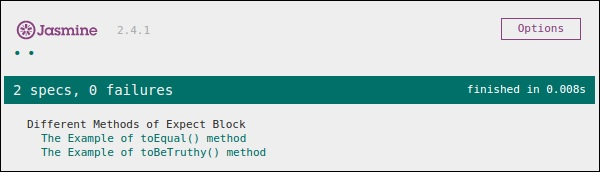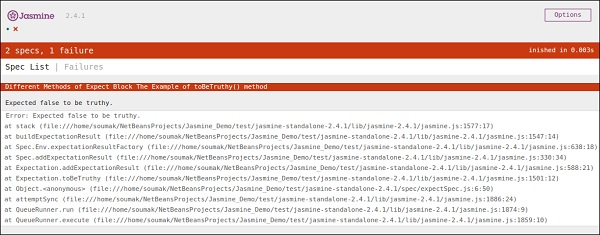# JasmineJS - Boolean Check

Apart from equality check, Jasmine provides some methods to check Boolean conditions too. Following are the methods that help us check Boolean conditions.

## ToBeTruthy()

This Boolean matcher is used in Jasmine to check whether the result is equal to true or false.

The following example will help us understand the working principle of the toBeTruthy() function.

### ExpectSpec.js

```describe("Different Methods of Expect Block",function () {
it("The Example of toBeTruthy() method",function () {
expect(expectexam.exampleoftrueFalse(5)).toBeTruthy();
});
});
```

### Expectexam.js

```window.expectexam = {
exampleoftrueFalse: function (num) {
if(num < 10)
return true;
else
return false;
},
};
```

As we are passing number 5, which is smaller than 10, this test case will pass and give us the following output.If we pass a number which is larger than 10, then this green test will change to red. In the second screenshot, you can see that on passing some value which is greater than 10, the expected test case fails and generates red output stating that “Expected false to be truthy”.## toBeFalsy()

toBeFalsy() also works the same way as toBeTruthy() method. It matches the output to be false whereas toBeTruthy matches the output to be true. The following example will help you understand the basic working principles of toBeFalsy().

### ExpectSpec.js

```describe("Different Methods of Expect Block",function() {
it("The Example of toBeTruthy() method",function () {
expect(expectexam.exampleoftrueFalse(15)).toBeFalsy();
});
});
```

### Expectexam.js

```window.expectexam = {
exampleoftrueFalse: function (num) {
if(num < 10)
Return true;
else
return false;
},
};
```

The above code will pass the Jasmine test case as we are passing value more than 10 and expected the output to be false. Hence, the browser will show us a green sign which means it has passed.